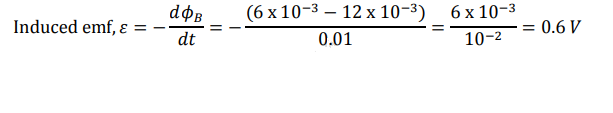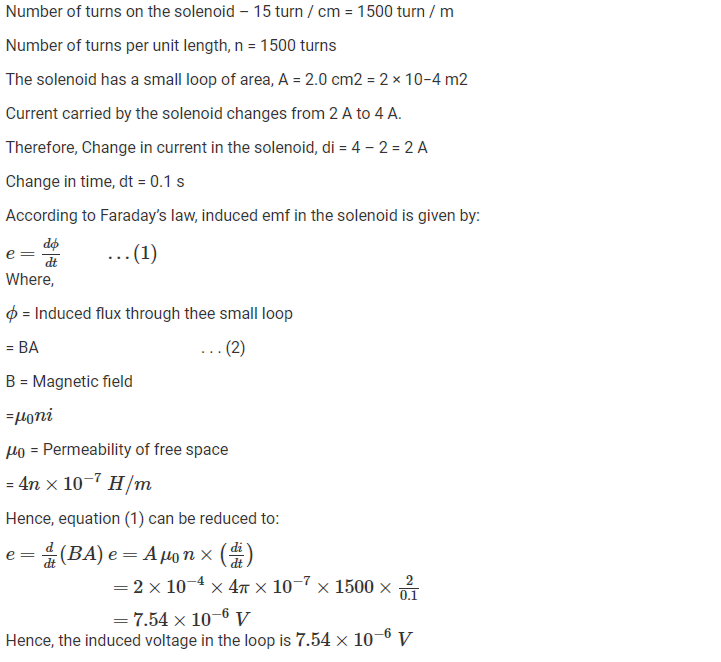# Magnetic Induction Formula

Magnetic induction was discovered by Michael Faraday in 1831. Later Maxwell described it mathematically and it came to be known as Faraday’s law of induction. Faraday had performed three experiments to understand electromagnetic induction. Then Faraday’s law became crucial to understand induction which now has several practical applications like in generators, transformers, etc.

## Law of Magnetic Induction

Magnetic induction, also called electromagnetic induction refers to the production of voltage (or EMF) across an electrical conductor placed inside a varying magnetic field. According to Faraday’s law, for a closed circuit, the induced electromotive force is equal to the rate of change of the magnetic flux enclosed by the circuit. To know more about magnetic induction, visit electromagnetic induction.

### Formula For Magnetic Induction

From Faraday’s law, the EMF induced in a closed circuit is given by –$\epsilon = \frac{d\Phi_{b} }{dt}$

Here,$\Phi _{b}$ is the magnetic flux, t is the time and$\epsilon$ is the EMF induced.

Note:$\Phi _{b} = \oint\vec{B}\cdot d\vec{s}$

Where, B = magnetic field and “ds” is a very small area.

In a coil of wire with N turns, the EMF will be-$\epsilon = N\frac{d\Phi_{b} }{dt}$

Later, according to Lenz law, Faraday’s equation was modified accordingly which is now given by-$\epsilon = N\frac{d\Phi_{b} }{dt}$

Now, this equation determines the direction of induced current and follows the law of conservation of energy.

### For a moving conductor, the EMF is given by:$\epsilon = Blv\, sin\Theta$

Where, l = length of the conductor, v = velocity of the conductor and θ is the angle between the magnetic field and the direction of motion.

An example related to the magnetic induction is given below for better understanding.

### Solved Examples

Example 1:

Calculate the induced EMF if the magnetic flux linked with a coil changes from 12 x 10-3 Wb to 6 x 10-3 Wb in 0.01 second.

Solution:Example 2:

A long solenoid having 15 turns per cm and a small loop area of 2 cm2 is placed in a solenoid to its axis. Find the induced emf in the loop while the current carried by the solenoid is changing steadily from 2.0 A to 4.0 A in 0.1 s.Stay tuned with BYJU’S for more such interesting articles. Also, register to “BYJU’S – The Learning App” for loads of interactive, engaging Physics-related videos and an unlimited academic assist.